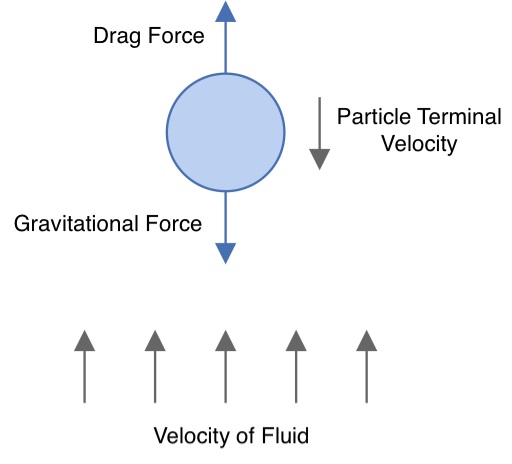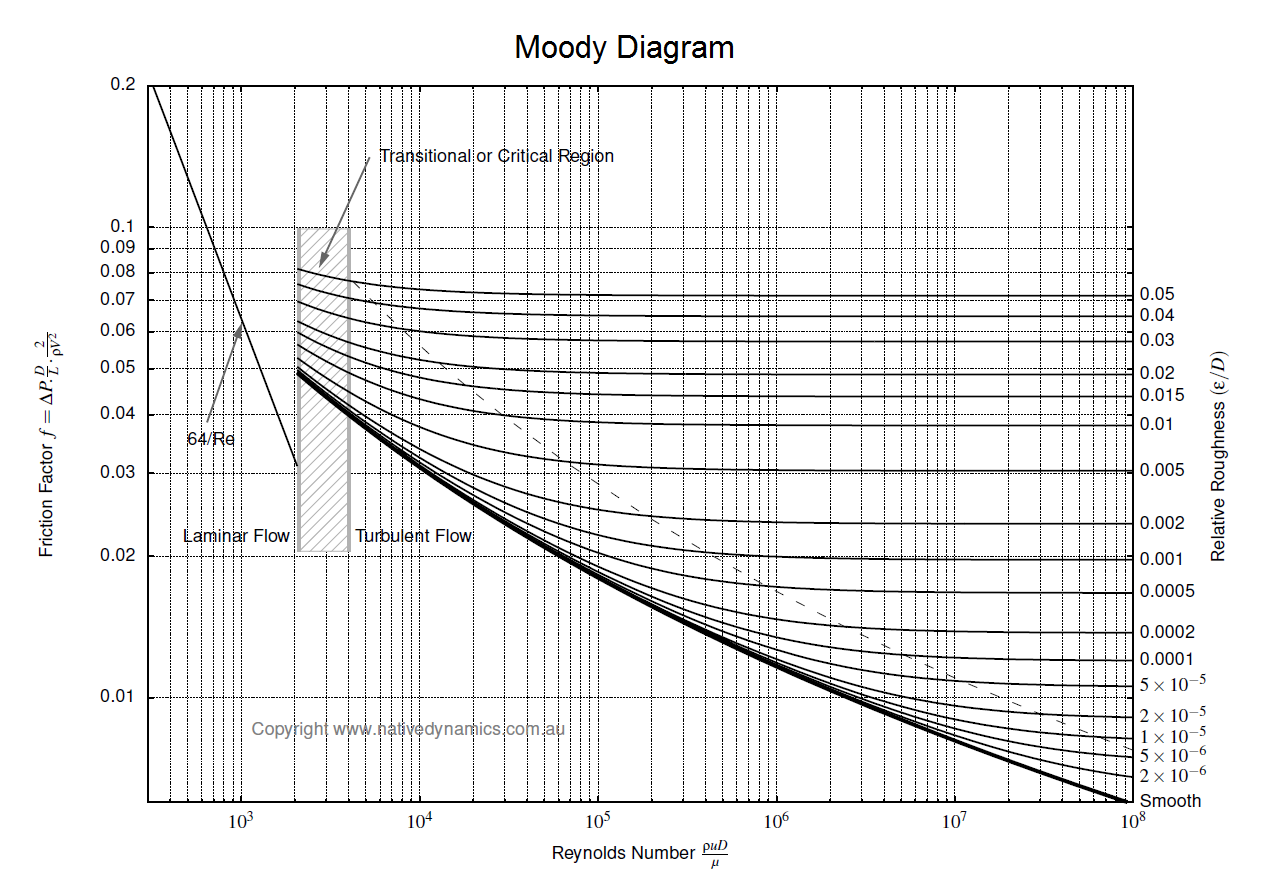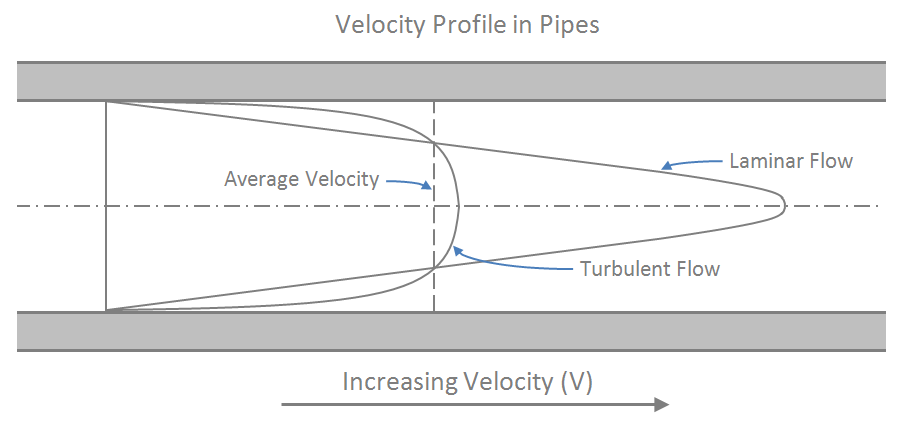f
Neutrium

# Flow Regime

Terminal Velocity of Particles for Gravity SeparationThere are many operations in which two phases must be separated. These separations may be gas-liquid, gas-solid, liquid-liquid or liquid-solid, with several factors such as relative densities, gravity, fluid velocities and the shape of particles and/or droplets influence the phase separability. In this article we present the fundamentals of these separations and provide the Stoke’s, Intermediate and Newton’s formulae for calculating the terminal velocities of settling particles to analyse separation systems.

Pressure Loss in PipeTo determine the pressure loss or flow rate through pipe knowledge of the friction between the fluid and the pipe is required. This article describes how to incorporate friction into pressure loss or fluid flow calculations. It also outlines several methods for determining the Darcy friction factor for rough and smooth pipes in both the turbulent and laminar flow regime. Finally this article discusses which correlation for pressure loss in pipe is the most appropriate.

Reynolds NumberThe Reynolds number is dimensionless and describes the ratio of inertial forces to viscous forces in a flowing fluid. It is used in many fluid flow correlations and is used to describe the boundaries of fluid flow regimes (laminar, transitional and turbulent). This article will show you how to calculate and interpret the Reynolds number.